# C++虚函数及虚函数表解析

• 对 C++ 多态内部机制了解的渴望。
• 眼过千遍，不如手过一遍。
• 整理成文，帮助自己记忆；不求帮到他人，只求不会误导。

## 1. 背景知识（一些基本概念）

C++ "虚函数"的存在是为了实现面向对象中的"多态"，即父类类别的指针（或者引用）指向其子类的实例，然后通过父类的指针（或者引用）调用实际子类的成员函数。 通过动态赋值，实现调用不同的子类的成员函数（动态绑定）。正是因为这种机制，把析构函数声明为"虚函数"可以防止在内存泄露。

```#include <iostream>
using namespace std;

class base_class
{
public:
base_class()
{
}
virtual ~base_class()
{
}

int normal_func()
{
cout << "This is  base_class's normal_func()" << endl;
return 0;
}
virtual int virtual_fuc()
{
cout << "This is  base_class's virtual_fuc()" << endl;
return 0;
}

};

class drived_class1 : public base_class
{
public:
drived_class1()
{
}
virtual ~drived_class1()
{
}

int normal_func()
{
cout << "This is  drived_class1's normal_func()" << endl;
return 0;
}
virtual int virtual_fuc()
{
cout << "This is  drived_class1's virtual_fuc()" << endl;
return 0;
}
};

class drived_class2 : public base_class
{
public:
drived_class2()
{
}
virtual ~drived_class2()
{
}

int normal_func()
{
cout << "This is  drived_class2's normal_func()" << endl;
return 0;
}
virtual int virtual_fuc()
{
cout << "This is  drived_class2's virtual_fuc()" << endl;
return 0;
}
};

int main()
{
base_class * pbc = NULL;
base_class bc;
drived_class1 dc1;
drived_class2 dc2;

pbc = &bc;
pbc->normal_func();
pbc->virtual_fuc();

pbc = &dc1;
pbc->normal_func();
pbc->virtual_fuc();

pbc = &dc2;
pbc->normal_func();
pbc->virtual_fuc();
return 0;

}
```

```This is  base_class's normal_func()
This is  base_class's virtual_fuc()
This is  base_class's normal_func()
This is  drived_class1's virtual_fuc()
This is  base_class's normal_func()
This is  drived_class2's virtual_fuc()
```

```// 无实现体
virtual int virtual_fuc() = 0;
```

## 2. 无继承时的虚函数表

```#include <iostream>
using namespace std;

class base_class
{
public:
virtual void v_func1()
{
cout << "This is base_class's v_func1()" << endl;
}
virtual void v_func2()
{
cout << "This is base_class's v_func2()" << endl;
}
virtual void v_func3()
{
cout << "This is base_class's v_func3()" << endl;
}
};

int main()
{
// 查看 base_class 的虚函数表
base_class bc;
cout << "base_class 的虚函数表首地址为：" << (int*)&bc << endl; // 虚函数表地址存在对象的前四个字节
cout << "base_class 的 第一个函数首地址：" << (int*)*(int*)&bc+0 << endl; // 指针运算看不懂？没关系，一会解释给你听
cout << "base_class 的 第二个函数首地址：" << (int*)*(int*)&bc+1 << endl;
cout << "base_class 的 第三个函数首地址：" << (int*)*(int*)&bc+2 << endl;
cout << "base_class 的 结束标志: " << *((int*)*(int*)&bc+3) << endl;

// 通过函数指针调用函数，验证正确性
typedef void(*func_pointer)(void);
func_pointer fp = NULL;
fp = (func_pointer)*((int*)*(int*)&bc+0); // v_func1()
fp();
fp = (func_pointer)*((int*)*(int*)&bc+1); // v_func2()
fp();
fp = (func_pointer)*((int*)*(int*)&bc+2); // v_func3()
fp();
return 0;
}
```

```base_class 的虚函数表首地址为：0x22ff0c
base_class 的 第一个函数首地址：0x472c98
base_class 的 第二个函数首地址：0x472c9c
base_class 的 第三个函数首地址：0x472ca0
base_class 的虚函数表结束标志: 0
This is base_class's v_func1()
This is base_class's v_func2()
This is base_class's v_func3()
```

• `&bc` ：获得 bc 对象的地址。
• `(int)&bc`: 类型转换，获得虚函数表的首地址。这里使用 `int` 的原因是函数指针的大小的 4byte，使用 `int*` 可以使得他们每次的偏移量保持一致（ `sizeof(int*) = 4` ，32-bit 机器）。
• `(int)&bc` ：解指针引用，获得虚函数表。
• `(int)(int*)&bc+0` ：和上面相同的类型转换，获得虚函数表的第一个虚函数地址。
• `(int)(int*)&bc+1` ：同上，获得第二个函数地址。
• `(int)(int*)&bc+2` ：同上，获得第三个函数地址。
• `((int)(int)&bc+3)` ：获得虚函数表的结束标志，所以这里我解引用了。和我们使用链表的情况是一样的，虚函数表当然也需要一个结束标志。
• `typedef void(*func_pointer)(void)` ：定义一个函数指针，参数和返回值都是 void。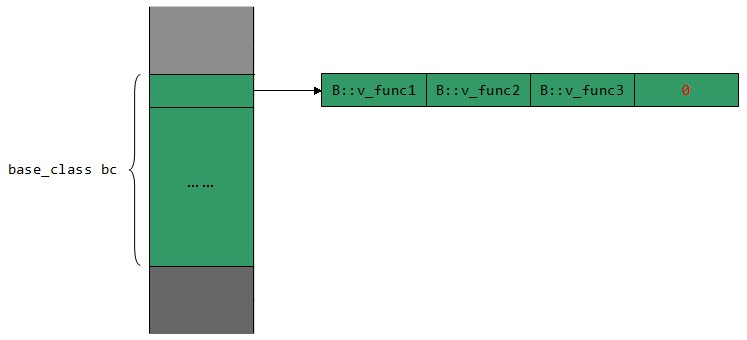## 3. 单一继承下的虚函数表

### 3.1. 子类没有重写父类的虚函数

```#include <iostream>
using namespace std;

class base_class
{
public:
virtual void v_func1()
{
cout << "This is base_class's v_func1()" << endl;
}
virtual void v_func2()
{
cout << "This is base_class's v_func2()" << endl;
}
virtual void v_func3()
{
cout << "This is base_class's v_func3()" << endl;
}
};
class dev_class : public base_class
{
public:
virtual void v_func4()
{
cout << "This is dev_class's v_func4()" << endl;
}
virtual void v_func5()
{
cout << "This is dev_class's v_func5()" << endl;
}
};

int main()
{
// 查看 dev_class 的虚函数表
dev_class dc;
cout << "dev_class 的虚函数表首地址为：" << (int*)&dc << endl;
cout << "dev_class 的 第一个函数首地址：" << (int*)*(int*)&dc+0 << endl;
cout << "dev_class 的 第二个函数首地址：" << (int*)*(int*)&dc+1 << endl;
cout << "dev_class 的 第三个函数首地址：" << (int*)*(int*)&dc+2 << endl;
cout << "dev_class 的 第四个函数首地址：" << (int*)*(int*)&dc+3 << endl;
cout << "dev_class 的 第五个函数首地址：" << (int*)*(int*)&dc+4 << endl;
cout << "dev_class 的虚函数表结束标志: " << *((int*)*(int*)&dc+5) << endl;
// 通过函数指针调用函数，验证正确性
typedef void(*func_pointer)(void);
func_pointer fp = NULL;
for (int i=0; i<5; i++) {
fp = (func_pointer)*((int*)*(int*)&dc+i);
fp();
}
return 0;
}
```

```dev_class 的虚函数表首地址为：0x22ff0c
dev_class 的 第一个函数首地址：0x472d10
dev_class 的 第二个函数首地址：0x472d14
dev_class 的 第三个函数首地址：0x472d18
dev_class 的 第四个函数首地址：0x472d1c
dev_class 的 第五个函数首地址：0x472d20
dev_class 的虚函数表结束标志: 0
This is base_class's v_func1()
This is base_class's v_func2()
This is base_class's v_func3()
This is dev_class's v_func4()
This is dev_class's v_func5()
```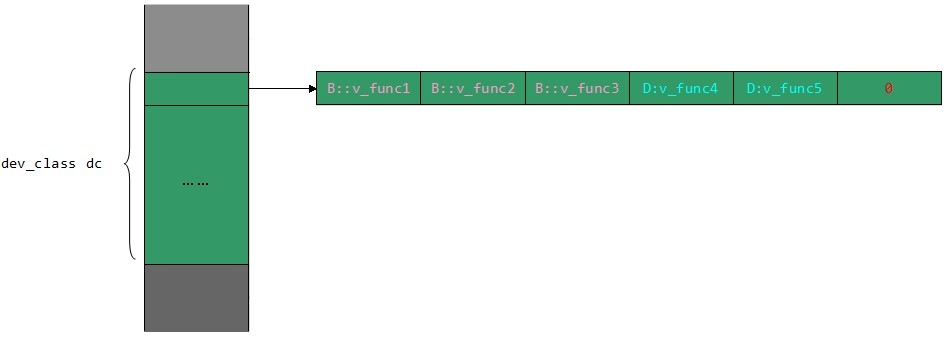### 3.2. 子类有重写父类的虚函数

```#include <iostream>
using namespace std;

class base_class
{
public:
virtual void v_func1()
{
cout << "This is base_class's v_func1()" << endl;
}
virtual void v_func2()
{
cout << "This is base_class's v_func2()" << endl;
}
virtual void v_func3()
{
cout << "This is base_class's v_func3()" << endl;
}
};
class dev_class : public base_class
{
public:
virtual void v_func1()
{
cout << "This is dev_class's v_func1()" << endl;
}
virtual void v_func2()
{
cout << "This is dev_class's v_func2()" << endl;
}
virtual void v_func4()
{
cout << "This is dev_class's v_func4()" << endl;
}
virtual void v_func5()
{
cout << "This is dev_class's v_func5()" << endl;
}
};

int main()
{
// 查看 dev_class 的虚函数表
dev_class dc;
cout << "dev_class 的虚函数表首地址为：" << (int*)&dc << endl;
cout << "dev_class 的 第一个函数首地址：" << (int*)*(int*)&dc+0 << endl;
cout << "dev_class 的 第二个函数首地址：" << (int*)*(int*)&dc+1 << endl;
cout << "dev_class 的 第三个函数首地址：" << (int*)*(int*)&dc+2 << endl;
cout << "dev_class 的 第四个函数首地址：" << (int*)*(int*)&dc+3 << endl;
cout << "dev_class 的 第五个函数首地址：" << (int*)*(int*)&dc+4 << endl;
cout << "dev_class 的虚函数表结束标志: " << *((int*)*(int*)&dc+5) << endl;
// 通过函数指针调用函数，验证正确性
typedef void(*func_pointer)(void);
func_pointer fp = NULL;
for (int i=0; i<5; i++) {
fp = (func_pointer)*((int*)*(int*)&dc+i);
fp();
}
return 0;
}
```

```dev_class 的虚函数表首地址为：0x22ff0c
dev_class 的 第一个函数首地址：0x472d50
dev_class 的 第二个函数首地址：0x472d54
dev_class 的 第三个函数首地址：0x472d58
dev_class 的 第四个函数首地址：0x472d5c
dev_class 的 第五个函数首地址：0x472d60
dev_class 的虚函数表结束标志: 0
This is dev_class's v_func1()
This is dev_class's v_func2()
This is base_class's v_func3()
This is dev_class's v_func4()
This is dev_class's v_func5()
```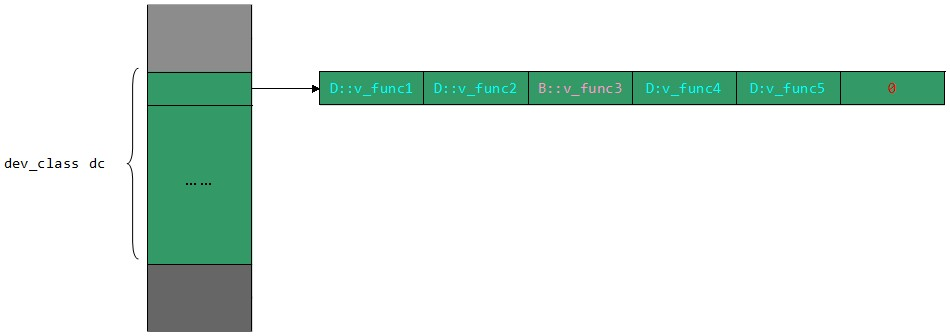```virtual void v_func1()
{
base_class::v_func1();
cout << "This is dev_class's v_func1()" << endl;
}
```

base_class::v_func1，会不会有错呢？答案是没错的，可以正确的调用！不是覆盖了吗？dev_class 已经不知道 base_class::v_func1 的指针了，怎么调用的呢？

## 4. 多重继承下的虚函数表

### 4.1. 子类没有重写父类的虚函数

```#include <iostream>
using namespace std;

class base_class1
{
public:
virtual void bc1_func1()
{
cout << "This is bc1_func1's v_func1()" << endl;
}
};

class base_class2
{
public:
virtual void bc2_func1()
{
cout << "This is bc2_func1's v_func1()" << endl;
}
};

class dev_class : public base_class1, public base_class2
{
public:
virtual void dc_func1()
{
cout << "This is dc_func1's dc_func1()" << endl;
}
};

int main()
{
dev_class dc;
cout << "dc 的虚函数表 bc1_vt 地址：" << (int*)&dc << endl;
cout << "dc 的虚函数表 bc1_vt 第一个虚函数地址：" << (int*)*(int*)&dc+0 << endl;
cout << "dc 的虚函数表 bc1_vt 第二个虚函数地址：" << (int*)*(int*)&dc+1 << endl;
cout << "dc 的虚函数表 bc1_vt 结束标志：" << *((int*)*(int*)&dc+2) << endl;
cout << "dc 的虚函数表 bc2_vt 地址：" << (int*)&dc+1 << endl;
cout << "dc 的虚函数表 bc2_vt 第一个虚函数首地址：：" << (int*)*((int*)&dc+1)+0 << endl;
cout << "dc 的虚函数表 bc2_vt 结束标志：" << *((int*)*((int*)&dc+1)+1) << endl;
// 通过函数指针调用函数，验证正确性
typedef void(*func_pointer)(void);
func_pointer fp = NULL;
// bc1_vt
fp = (func_pointer)*((int*)*(int*)&dc+0);
fp();
fp = (func_pointer)*((int*)*(int*)&dc+1);
fp();
// bc2_vt
fp = (func_pointer)*(((int*)*((int*)&dc+1)+0));
fp();
return 0;
}
```

```dc 的虚函数表 bc1_vt 地址：0x22ff08
dc 的虚函数表 bc1_vt 第一个虚函数地址：0x472d38
dc 的虚函数表 bc1_vt 第二个虚函数地址：0x472d3c
dc 的虚函数表 bc1_vt 结束标志：-4
dc 的虚函数表 bc2_vt 地址：0x22ff0c
dc 的虚函数表 bc2_vt 第一个虚函数首地址：：0x472d48
dc 的虚函数表 bc2_vt 结束标志：0
This is bc1_func1's v_func1()
This is dc_func1's dc_func1()
This is bc2_func1's v_func1()
```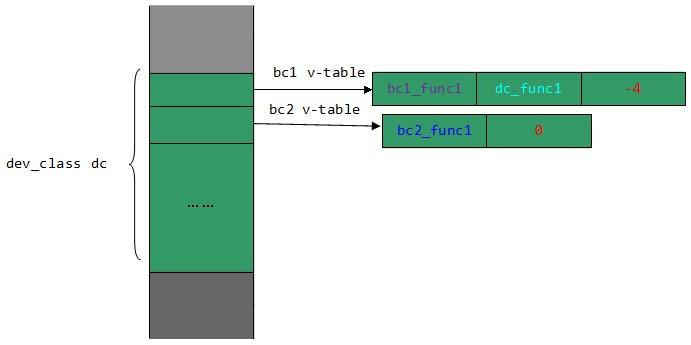### 4.2. 子类重写了父类的虚函数

```#include <iostream>
using namespace std;

class base_class1
{
public:
virtual void bc1_func1()
{
cout << "This is base_class1's bc1_func1()" << endl;
}
virtual void bc1_func2()
{
cout << "This is base_class1's bc1_func2()" << endl;
}
};

class base_class2
{
public:
virtual void bc2_func1()
{
cout << "This is base_class2's bc2_func1()" << endl;
}
virtual void bc2_func2()
{
cout << "This is base_class2's bc2_func2()" << endl;
}
};

class dev_class : public base_class1, public base_class2
{
public:
virtual void bc1_func1()
{
cout << "This is dev_class's bc1_func1()" << endl;
}
virtual void bc2_func1()
{
cout << "This is dev_class's bc2_func1()" << endl;
}
virtual void dc_func1()
{
cout << "This is dev_class's dc_func1()" << endl;
}
};

int main()
{
dev_class dc;
cout << "dc 的虚函数表 bc1_vt 地址：" << (int*)&dc << endl;
cout << "dc 的虚函数表 bc1_vt 第一个虚函数地址：" << (int*)*(int*)&dc+0 << endl;
cout << "dc 的虚函数表 bc1_vt 第二个虚函数地址：" << (int*)*(int*)&dc+1 << endl;
cout << "dc 的虚函数表 bc1_vt 第三个虚函数地址：" << (int*)*(int*)&dc+2 << endl;
cout << "dc 的虚函数表 bc1_vt 第四个虚函数地址：" << (int*)*(int*)&dc+3 << endl;
cout << "dc 的虚函数表 bc1_vt 结束标志：" << *((int*)*(int*)&dc+4) << endl;
cout << "dc 的虚函数表 bc2_vt 地址：" << (int*)&dc+1 << endl;
cout << "dc 的虚函数表 bc2_vt 第一个虚函数首地址：：" << (int*)*((int*)&dc+1)+0 << endl;
cout << "dc 的虚函数表 bc2_vt 第二个虚函数首地址：：" << (int*)*((int*)&dc+1)+1 << endl;
cout << "dc 的虚函数表 bc2_vt 结束标志：" << *((int*)*((int*)&dc+1)+2) << endl;
// 通过函数指针调用函数，验证正确性
typedef void(*func_pointer)(void);
func_pointer fp = NULL;
// bc1_vt
fp = (func_pointer)*((int*)*(int*)&dc+0);
fp();
fp = (func_pointer)*((int*)*(int*)&dc+1);
fp();
fp = (func_pointer)*((int*)*(int*)&dc+2);
fp();
fp = (func_pointer)*((int*)*(int*)&dc+3);
fp();
// bc2_vt
fp = (func_pointer)*(((int*)*((int*)&dc+1)+0));
fp();
fp = (func_pointer)*(((int*)*((int*)&dc+1)+1));
fp();
return 0;
}
```

```dc 的虚函数表 bc1_vt 地址：0x22ff08
dc 的虚函数表 bc1_vt 第一个虚函数地址：0x472e28
dc 的虚函数表 bc1_vt 第二个虚函数地址：0x472e2c
dc 的虚函数表 bc1_vt 第三个虚函数地址：0x472e30
dc 的虚函数表 bc1_vt 第四个虚函数地址：0x472e34
dc 的虚函数表 bc1_vt 结束标志：-4
dc 的虚函数表 bc2_vt 地址：0x22ff0c
dc 的虚函数表 bc2_vt 第一个虚函数首地址：：0x472e40
dc 的虚函数表 bc2_vt 第一个虚函数首地址：：0x472e44
dc 的虚函数表 bc2_vt 结束标志：0
This is dev_class's bc1_func1()
This is base_class1's bc1_func2()
This is dev_class's bc2_func1()
This is dev_class's dc_func1()
This is dev_class's bc2_func1()
This is base_class2's bc2_func2()
```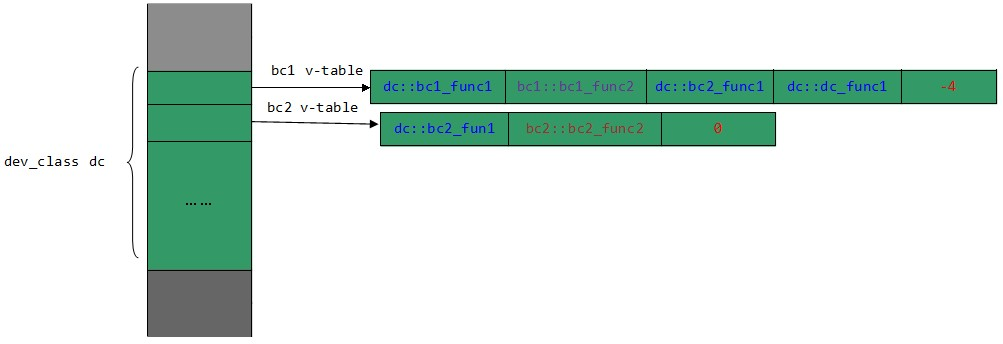• 实现方式是不一样的，但是它们的实现结果是一样的（多态）。
• 无论你了解虚函数表或者不了解虚函数表，我相信你都很少会用到它。但是当你了解了它的实现机制之后，你再去看多态， 再去写虚函数的时候[作为你一个coder]，相信你的感觉是不一样的。你会感觉很透彻，不会有丝毫的犹豫。
• 学习编译器这种处理问题的方式（思想），这才是最重要的。[好像扯远了]。

• 访问权限是怎么实现的？编译器怎么知道哪些函数是 public，哪些是 protected ？
• 虚函数调用是通过虚函数表实现的，那么非虚成员函数存放在哪里？是怎么实现的呢？
• 类的成员存放在什么位置？怎么继承的呢？[这是对象布局问题，=.=]

2012.07.20 update：

• 本文只针对 Windows 7 Code::blocks 10.05 进行测试和讲解；
• 不同的编译器实现方式可能不同，比如 VS2010 和 CB 10.05 就有些不同，感兴趣的朋友可自行测试。

First created: 2012-07-11 07:02:00
Last updated: 2022-12-11 Sun 12:49
Power by Emacs 27.1 (Org mode 9.4.4)